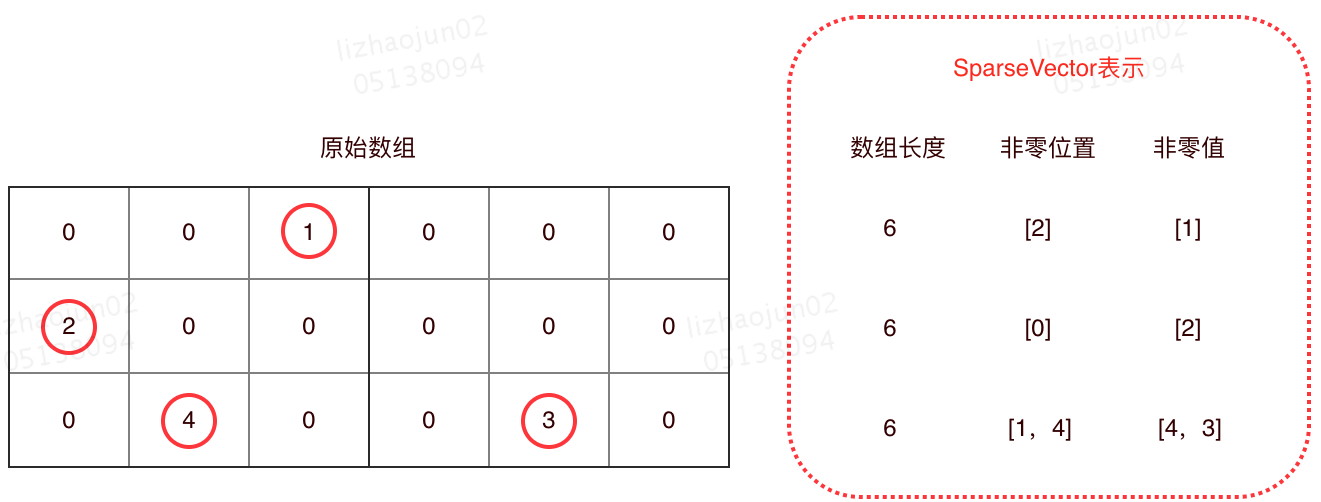# XGBoost 缺失值引发的问题及其深度分析## 1. 背景

XGBoost 模型作为机器学习中的一大“杀器”，被广泛应用于数据科学竞赛和工业领域，XGBoost 官方也提供了可运行于各种平台和环境的对应代码，如适用于 Spark 分布式训练的 XGBoost on Spark。然而，在 XGBoost on Spark 的官方实现中，却存在一个因 XGBoost 缺失值和 Spark 稀疏表示机制而带来的不稳定问题。

## 3. XGBoost on Spark 源码中缺失值引入的不稳定问题

Spark ML 中还有隐藏的缺失值处理逻辑：SparseVector，即稀疏向量。

SparseVector 和 DenseVector 都用于表示一个向量，两者之间仅仅是存储结构的不同。

SparseVector 的存储方式是：仅仅记录所有非 0 值，忽略掉所有 0 值。具体来说，用一个数组记录所有非 0 值的位置，另一个数组记录上述位置所对应的数值。有了上述两个数组，再加上当前向量的总长度，即可将原始的数组还原回来。

SparseVector 存储示例见下图：private[feature] def assemble(vv: Any*): Vector = {    val indices = ArrayBuilder.make[Int]    val values = ArrayBuilder.make[Double]    var cur = 0    vv.foreach {      case v: Double =>         //0 不进行保存        if (v != 0.0) {           indices += cur          values += v        }        cur += 1      case vec: Vector =>        vec.foreachActive { case (i, v) =>           //0 不进行保存          if (v != 0.0) {             indices += cur + i            values += v          }        }        cur += vec.size      case null =>        throw new SparkException("Values to assemble cannot be null.")      case o =>        throw new SparkException(s"$o of type${o.getClass.getName} is not supported.")    }    Vectors.sparse(cur, indices.result(), values.result()).compressed    }

    val instances: RDD[XGBLabeledPoint] = dataset.select(      col($(featuresCol)), col($(labelCol)).cast(FloatType),      baseMargin.cast(FloatType),      weight.cast(FloatType)    ).rdd.map { case Row(features: Vector, label: Float, baseMargin: Float, weight: Float) =>      val (indices, values) = features match {         //SparseVector 格式，仅仅将非 0 的值放入 XGBoost 计算        case v: SparseVector => (v.indices, v.values.map(_.toFloat))         case v: DenseVector => (null, v.values.map(_.toFloat))      }      XGBLabeledPoint(label, indices, values, baseMargin = baseMargin, weight = weight)    }

XGBoost on Spark 将 SparseVector 中的 0 值作为缺失值为什么会引入不稳定的问题呢

  /**   * Returns a vector in either dense or sparse format, whichever uses less storage.   */  @Since("2.0.0")  def compressed: Vector = {    val nnz = numNonzeros    // A dense vector needs 8 * size + 8 bytes, while a sparse vector needs 12 * nnz + 20 bytes.    if (1.5 * (nnz + 1.0) < size) {      toSparse    } else {      toDense    }  }

## 4. 问题解决

   val instances: RDD[XGBLabeledPoint] = dataset.select(      col($(featuresCol)), col($(labelCol)).cast(FloatType),      baseMargin.cast(FloatType),      weight.cast(FloatType)    ).rdd.map { case Row(features: Vector, label: Float, baseMargin: Float, weight: Float) =>       // 这里需要对原来代码的返回格式进行修改      val values = features match {         //SparseVector 的数据，先转成 Dense        case v: SparseVector => v.toArray.map(_.toFloat)         case v: DenseVector => v.values.map(_.toFloat)      }      XGBLabeledPoint(label, null, values, baseMargin = baseMargin, weight = weight)    }

    /**     * Converts a [[Vector]] to a data point with a dummy label.     *     * This is needed for constructing a [[ml.dmlc.xgboost4j.scala.DMatrix]]     * for prediction.     */    def asXGB: XGBLabeledPoint = v match {      case v: DenseVector =>        XGBLabeledPoint(0.0f, null, v.values.map(_.toFloat))      case v: SparseVector =>         //SparseVector 的数据，先转成 Dense        XGBLabeledPoint(0.0f, null, v.toArray.map(_.toFloat))     }

https://tech.meituan.com/2019/08/15/problems-caused-by-missing-xgboost-values-and-their-in-depth-analysis.html